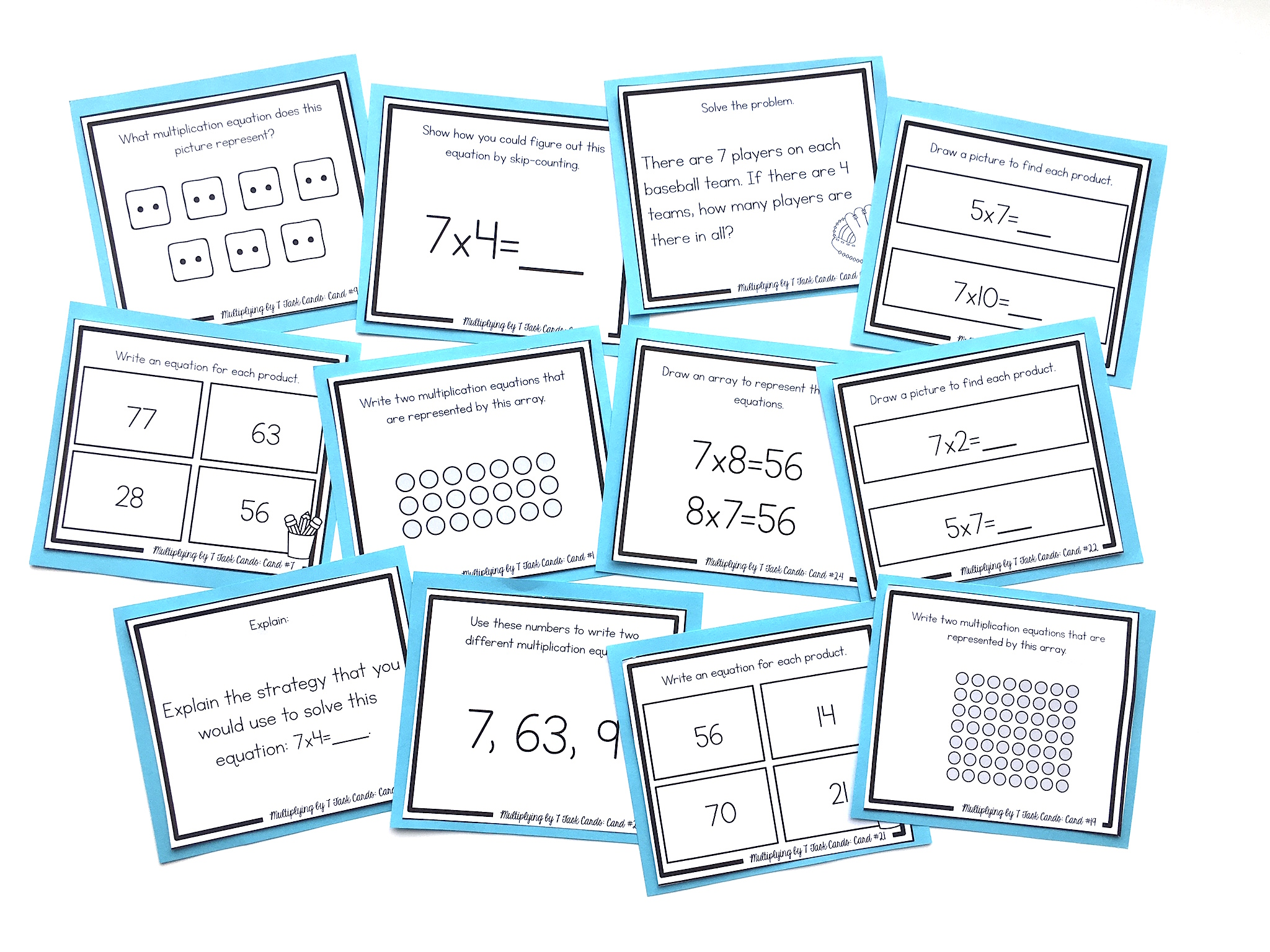# How to Teach the 6’s, 7’s, and 8’s Multiplication FactsThe 6’s, 7’s, and 8’s are typically some of the most difficult multiplication facts to learn. However, there is good news! If you are teaching your students using a strategic order of facts, they will only have a few facts left to learn for the 6’s, 7’s, and 8’s.

For these facts we can teach students how to use a known fact.

For example, for the equation 6×6, we could use a fact that we know like 5×6, and then just add one more group of 6.

For 7×7, we could use a fact that we know, such as 5×7. We know this is 35. Now we could add 2 more groups of 7 to make 49.

For 8×8, we could use a fact that we know, such as 9×8. We know that this is 72. Now we could SUBTRACT one group of 8 to make 64.

When I work with these facts with my students, I am ALWAYS asking this question: “What do you know,” because my goal is for students to begin asking themselves, “What do I know?” when they see a tricky equation.

Using known facts is a really important skill to learn. Although it may take a bit longer, it’s so important for students to learn that they can work with and manipulate numbers in order to solve an equation.

Ultimately, I prefer that my students memorize those really tough facts like 6×6, 6×7, 7×7, and 7×8. I work with these facts over and over and over again with a goal of students committing them to memory. However, those strategies are really important to fall back on.

NEXT STEPS

Reinforce the 6’s, 7’s, and 8’s multiplication facts with multiplication task cards. Students will learn conceptually through problem-solving, using arrays, strategic thinking, finding missing numbers, skip-counting, picture representations, and more. Find the 6’s task cards HERE, the 7’s HERE, and the 8’s HERE.OR FIND THE FULL BUNDLE OF MULTIPLICATION TASK CARDS HERE.